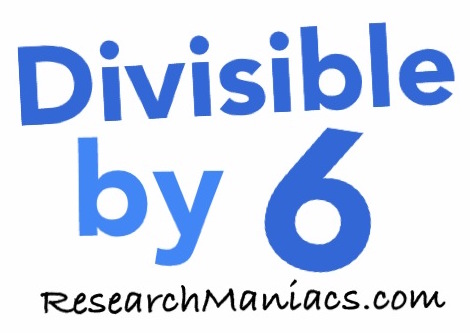Is 1 divisible by 6?The question we are solving here is: If we divide 1 by 6, will we get a whole number with no remainder? In other words, is 1 divisible by 6?

For 1 to be divisible by 6, both of the following two criteria must be met: First, 1 has to be an even number and second, the sum of its digits must be divisible by 3.

Looking at 1, you see that 1 is an odd number, which means that the first criteria is not met.

The sum of the digits in 1 is 1, which is not divisible by 3. Therefore, the second criteria is not met.

Thus, 1 is not divisible by 6 and the answer to the question "Is 1 divisible by 6?" is

NO

You may also be interested to know that if you divide 1 by 6, you get 0.17.

Divisible by 6?
Do you need to check another number? Enter a number below to see if it is divisible by 6.

Is 2 divisible by 6?
Can you do it on your own now? If not, go here for the next number on our list.